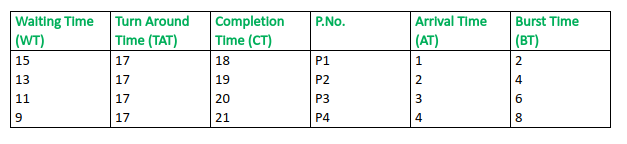# Longest Remaining Time First (LRTF) CPU Scheduling Algorithm

Prerequisite – Process Management | CPU Scheduling
This is a pre-emptive version of Longest Job First (LJF) scheduling algorithm. In this scheduling algorithm, we find the process with the maximum remaining time and then process it. We check for the maximum remaining time after some interval of time(say 1 unit each) to check if another process having more Burst Time arrived up to that time.

Procedure:

• Step-1: First, sort the processes in increasing order of their Arrival Time.
• Step-2: Choose the process having least arrival time but with most Burst Time. Then process it for 1 unit. Check if any other process arrives upto that time of execution or not.
• Step-3: Repeat the above both steps until execute all the processes.

Example-1: Consider the following table of arrival time and burst time for four processes P1, P2, P3 and P4.

```Process   Arrival time   Burst Time
P1            1 ms          2 ms
P2            2 ms          4 ms
P3            3 ms          6 ms
P4            4 ms          8 ms  ```

Working: (for input 1):

1. At t = 1, Available Process : P1. So, select P1 and execute 1 ms.
2. At t = 2, Available Process : P1, P2. So, select P2 and execute 1 ms (since BT(P1)=1 which is less than BT(P2) = 4)
3. At t = 3, Available Process : P1, P2, P3. So, select P3 and execute 1 ms (since, BT(P1) = 1 , BT(P2) = 3 , BT(P3) = 6).
4. Repeat the above steps until the execution of all processes.

Note that CPU will be idle for 0 to 1 unit time since there is no process available in the given interval.

Gantt chart will be as following below,Since, complietion time (CT) can be directly determined by Gantt chart, and

```Turn Around Time (TAT)
= (Complition Time) - (Arival Time)

Also, Waiting Time (WT)
= (Turn Around Time) - (Burst Time) ```

Therefore, final table look like,Output:

```Total Turn Around Time = 68 ms
So, Average Turn Around Time = 68/4 = 17.00 ms

And, Total Waiting Time = 48 ms
So Average Waiting Time = 48/4 = 12.00 ms ```

Example-2: Consider the following table of arrival time and burst time for four processes P1, P2, P3,P4 and P5.

```Process   Arrival time   Burst Time
P1            0 ms          2 ms
P2            0 ms          3 ms
P3            2 ms          2 ms
P4            3 ms          5 ms
P5            4 ms          4 ms ```

Similarly example-1, Gantt chart for this example,Since, complietion time (CT) can be directly determined by Gantt chart, and

```Turn Around Time (TAT)
= (Complition Time) - (Arival Time)

Also, Waiting Time (WT)
= (Turn Around Time) - (Burst Time) ```

Therefore, final table look like,Output:

```Total Turn Around Time = 61 ms
So, Average Turn Around Time = 61/5 = 12.20 ms

And, Total Waiting Time = 45 ms
So, Average Waiting Time = 45/5 = 9.00 ms ```My Personal Notes arrow_drop_upCheck out this Author's contributed articles.

If you like GeeksforGeeks and would like to contribute, you can also write an article using contribute.geeksforgeeks.org or mail your article to contribute@geeksforgeeks.org. See your article appearing on the GeeksforGeeks main page and help other Geeks.

Please Improve this article if you find anything incorrect by clicking on the "Improve Article" button below.